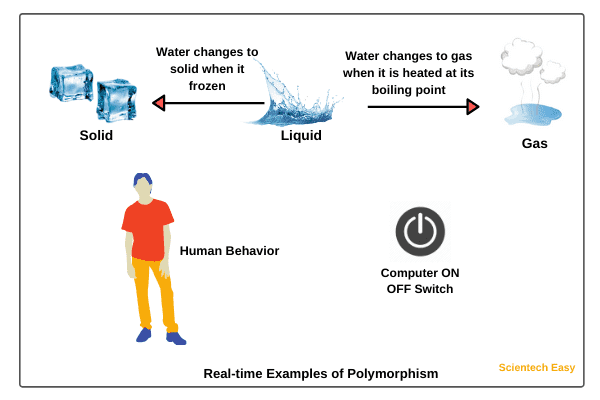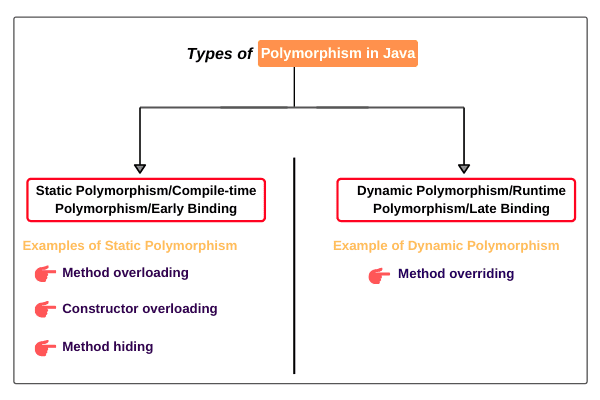# Polymorphism in Java | Compile Time, Runtime, Example

Polymorphism in java is one of the core concepts of object-oriented programming language (OOPs).

The word polymorphism is derived from two Greek words: poly and morphs. The word “poly” implies many and “morphs” means forms.

Therefore, polymorphism means “many forms”. That is one thing that can take many forms.

Polymorphism is a concept by which we can perform a single task in different ways. That is, when a single entity behaves differently in different cases, it is called polymorphism in Java.

We can achieve flexibility in our code using polymorphism because we can perform various operations by using methods with the same names according to requirements.

Let’s understand it with some realtime examples.

## Realtime Example of Polymorphism in Java

There are several real-time examples of polymorphism in the world.1. We all know that water is a liquid, but it changes to solid when it frozen, and it changes to a gas when it is heated at its boiling point.

2. The best example of polymorphism is human behavior. One person can have different behavior. For example, a person acts as an employee in the office, a customer in the shopping mall, a passenger in bus/train, a student in school, and a son at home.

3. We all use a single button to switch ON and OFF the computer.

4. A boy starts his love by saying the word “friendship” but the girl ends that love with the same word “friendship”. The girl says that we will be always good friends.

Here, the word “friendship” is the same but attitude is different. This beautiful concept is nothing but polymorphism.

## Types of Polymorphism in Java

Basically, there are two types of polymorphism in java. They are:

1. Static polymorphism
2. Dynamic polymorphismPolymorphism can be either static or dynamic depending on how the method is called in a class.

## Static Polymorphism in Java

A polymorphism that exhibited during compilation is called static polymorphism in java. In static polymorphism, the behavior of a method is decided at compile-time.

Hence, Java compiler binds method calls with method definition/body during compilation. Therefore, this type of polymorphism is also called compile-time polymorphism in Java.

Since binding is performed at compile-time, it is also known as early binding. Compile-time polymorphism can be achieved/implemented by method overloading in java.

Method overloading is a mechanism in which a class has multiple methods having the same name but different signatures. It is one of the ways that Java implements polymorphism.

## Java Compile-time Polymorphism Example Program

Let’s take an example program where we will implement static polymorphism. In this example program, we will create a class StaticPoly.

In this class, we will create two methods having the same name sum. Both of these methods will have different signatures. Let’s start coding.

Program source code 1:

```package staticPolymorphism;
public class StaticPoly
{
void sum(int x, int y)
{
int s = x + y;
System.out.println("Sum of two numbers: " +s);
}
void sum(int x, int y, int z)
{
int s = x + y + z;
System.out.println("Sum of three numbers: " +s);
}
public static void main(String[] args)
{
StaticPoly obj = new StaticPoly();
obj.sum(20, 10);
obj.sum(10, 20, 30);
}
}```
```Output:
Sum of two numbers: 30
Sum of three numbers: 60```

Explanation:

As you can observe in the preceding example program, the sum() method is overloaded two times because both methods’ signatures vary in the number of parameters.

The first sum() method accepts two parameters whereas, the second sum() method accepts three parameters.

When we will call the first sum() method using reference variable “obj” by passing two int type argument values, Java compiler binds the definition of sum(int x, int y) method with sum(20, 10) method during compilation and calls the appropriate method.

Hence, the sum of two numbers is displayed by invoking sum() method on the console.

Similarly, when we call the second sum() method by passing three int type argument values, Java compiler binds the definition of sum(int x, int y, int z) with sum(10, 20, 30) method during compilation, and calls a method of the sum of three numbers.

Thus, Java compiler matches the values passed to a method during compilation, binds method call with appropriate method definition, and calls the appropriate method.

In this way, compile-time polymorphism allows us to perform various operations by using multiple methods with the same name.

Key point:

Java compiler differentiates multiple methods having the same name by their signatures.

## Dynamic Polymorphism in Java

A polymorphism that is exhibited at runtime is called dynamic polymorphism in java. In dynamic polymorphism, the behavior of a method is decided at runtime,

therefore, the JVM (Java Virtual Machine) binds the method call with method definition/body at runtime and invokes the relevant method during runtime when the method is called.

This happens because objects are created at runtime and the method is called using an object of the class. The Java compiler has no awareness of the method to be called on an instance during compilation. Therefore, JVM invokes the relevant method during runtime.

Dynamic or runtime polymorphism can be achieved/implemented in java using method overriding. Method overriding is a mechanism where a method of Base class is overridden in the derived class to provide a more specific implementation.

The signature of method in both base and derived classes is the same but they only differ in their implementation.

Let’s take an example program where we will implement dynamic polymorphism by method overriding.

## Java Runtime Polymorphism Example Program

Let us consider two classes Base and Derived, as shown in the below code snippet. In both classes, we will declare a method named “calc” having the same signature.

Program source code 2:

```package dynamicPoly;
public class Base
{
void m1()
{
System.out.println("m1-Base");
}
}
public class Derived extends Base
{
void m1()
{
System.out.println("m1-Derived");
}
}
public class DynamicPoly
{
public static void main(String [] args)
{
Derived d = new Derived();
d.m1(); // Calling m1() method of class Derived.

Base b = new Base();
b.m1(); // Calling m1() method of class Base.
}
}```
```Output:
m1-Derived
m1-Base```

As you can observe in the above program, the class Base contains a method named m1. This m1() method is overridden in the derived class named Derived.

Since m1() method in both Base and Derived classes have the same name and signature, therefore, the Java compiler is unable to bind method calls with method definitions.

Hence, based on an object of a class, JVM decides to execute a suitable method at runtime. In this way, method overriding is one way to implement runtime polymorphism in java.

For a more detailed explanation of method overriding, go to this tutorial: Method overriding in Java

Hope that this tutorial has elaborated all the key points related to polymorphism in Java with example programs. I hope that you will have understood the types of polymorphism: compile-time and runtime and enjoyed example programs.